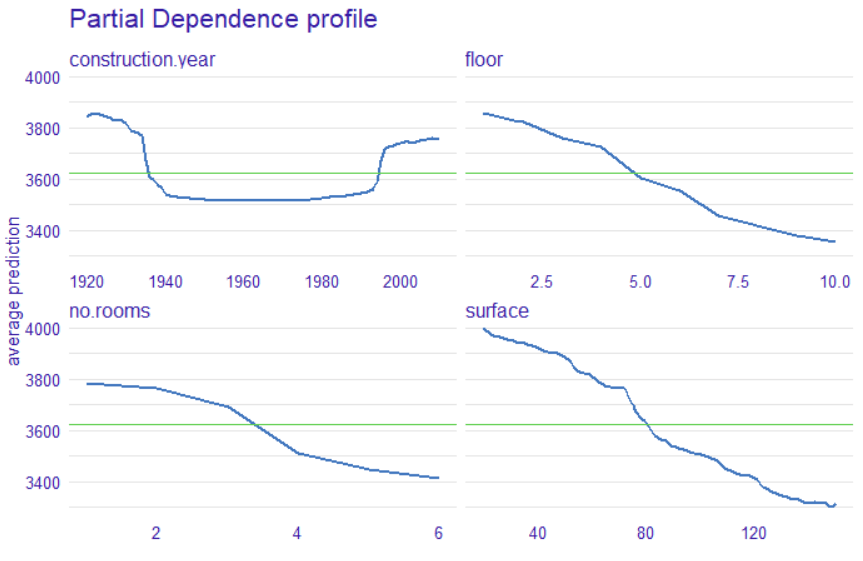Want to share your content on R-bloggers? click here if you have a blog, or here if you don't.

### vivo — variable importance via PDP oscillations

One of the many questions that are asked when analyzing a model is what variables are most important and how they impact the prediction.

We can consider several methods that depend on the type of model. The first one is the linear model, we can easily indicate the importance of the variables by looking at the coefficients and the significance of the statistical test. For models based on trees we can use a method based on using a calculation of the Gini impurity for each tree, then calculate an average.

For random forests, we can use the out-of-bag based method.

For other models, we can use model agnostic method — permutation base variable importance. You can read more about it in BASIC XAI with DALEX — Part 2: Permutation-based variable importance blog.

Now we will present another method for variable importance globally but also locally based on Partial Dependence Profiles (PDP) and Ceteris Paribus (CP) profiles respectively. We call this measure oscillations, it is implemented in R package vivo. Package is available on CRAN and GitHub.

#### How does it work?

We can see the fluctuation when we calculate and plot the profiles, be it PDP or CP. When this fluctuation is “large” it can mean that the importance of this variable is also large. When the profile is flat, close to the horizontal line, then the variable does not have much influence on the prediction. Observing such a dependence, we can build a measure on oscillations, i.e., we look at the change in profiles relative to a certain cutoff point. In the case of PDP profiles, this is the average response of the models and the measure is the area defined by this point and the profile. For local importance of variables (i.e., one observation), we can relate this baseline to two values. First, we can also use the average prediction for the whole sample, and we can use the prediction for the observation we are analyzing.

#### 3 steps to build a measure (in the global case)

How to build the model on which we present the methods — see here.

1. Calculate the PDP and plot it.
```pdp <- model_profile(explainer,
variables = c("construction.year",
"floor",
"no.rooms",
"surface")
)
plot(pdp)```

2. Define the base levelBase level as the mean value of the prediction for all observations

3. Calculate the painted area

```library(vivo)
measure <- global_variable_importance(pdp)
measure
variable_name  measure _label_model_
1 construction.year 117.4269        ranger
2             floor 172.2265        ranger
3          no.rooms 147.9695        ranger
4           surface 215.0675        ranger
plot(measure)```

The measures available in vivo allow you to specify the importance of variables, but also to identify variables where the change in prediction is the largest. In case of any questions or problems feel free to open issues at https://github.com/ModelOriented/vivo.

If you are interested in other posts about explainable, fair, and responsible ML, follow #ResponsibleML on Medium.

In order to see more R related content visit https://www.r-bloggers.comvivo — variable importance via PDP oscillations was originally published in ResponsibleML on Medium, where people are continuing the conversation by highlighting and responding to this story.